# For a crest vertical curve, with the following data, Station of BVC: 10+50 Station of Vertex:...

For a crest vertical curve, with the following data,

Station of BVC: 10+50

Station of Vertex: 18+00

Station of EVC: 20+50

Elevation of Vertex: 1000ft

a) At what station is the highest point located?

b) What is the elevation of the high point?

c) An information center is located at station 18+50.98, what is the elevation of the information center?

d) What is the AASHTO K-value for the vertical curve?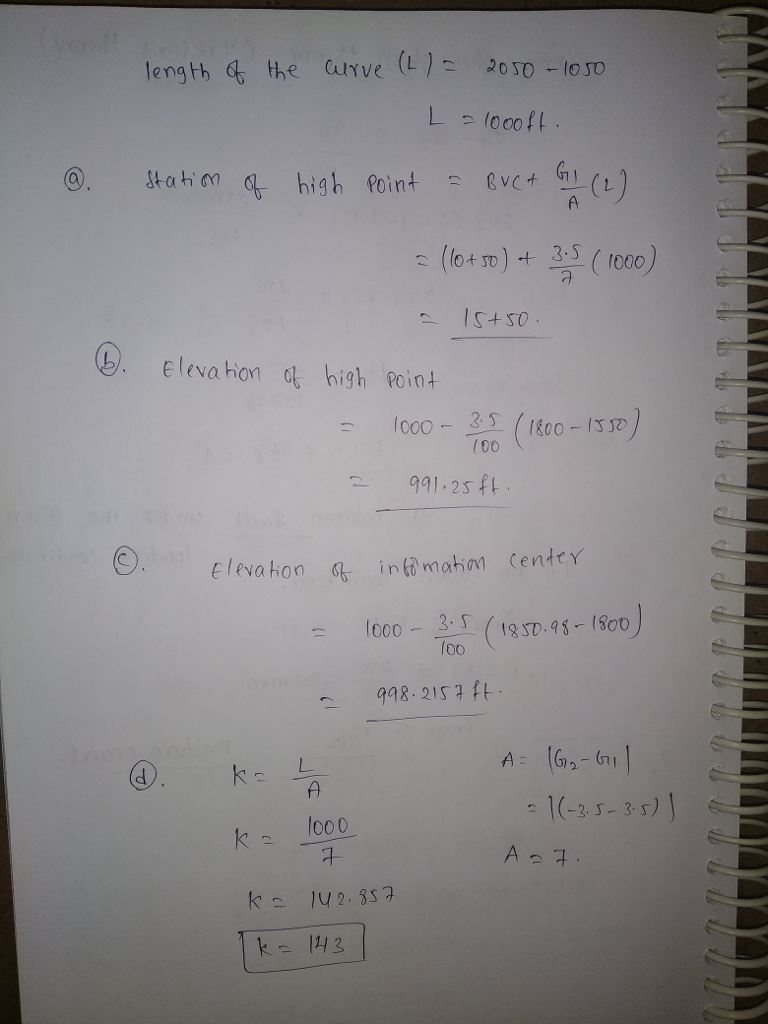#### Earn Coin

Coins can be redeemed for fabulous gifts.

Similar Homework Help Questions
• ### A crest vertical curve connects a +2% grade and a -2.05% grade. The PVI is at...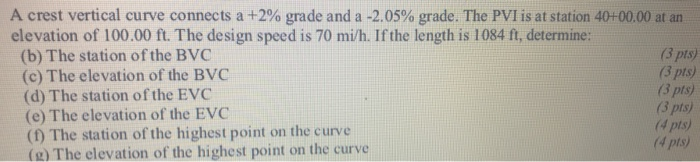A crest vertical curve connects a +2% grade and a -2.05% grade. The PVI is at station 40+00.00 at an elevation of 100.00 ft. The design speed is 70 mi/h. If the length is 1084 ft, determine: (b) The station of the BVC (3 pts (c) The elevation of the BVC (3 pts (d) The station of the EVC (3 pts) (e) The elevation of the EVC (3 pts) (1) The station of the highest point on the curve (4...

• ### Problem #3 (20 Points) Vertical Curve Problem (Use both Tangents) See Attached Vertical Curve Drawing Station...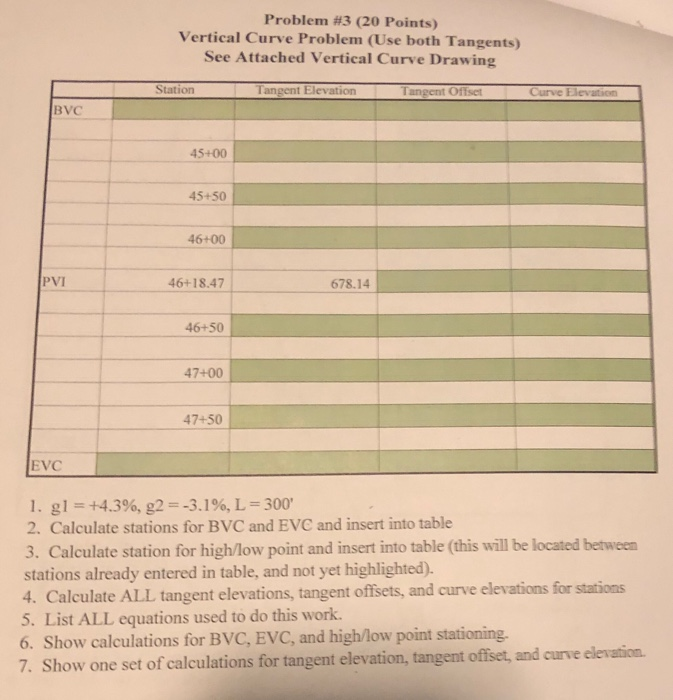Problem #3 (20 Points) Vertical Curve Problem (Use both Tangents) See Attached Vertical Curve Drawing Station Tangent Elevation Tangent Offset В VC 45+00 45+50 46+00 PVI 678.14 46+18.47 46+50 47+00 47+50 EVC I. gl=+4.3%, g2 =-3.196, L = 300, 2. Calculate stations for BVC and EVC and insert into table 3. Calculate station for high/low point and insert into table (this will be located between stations already entered in table, and not yet highlighted). 4. Calculate ALL tangent elevations, tangent...

• ### 9:381 LTE Problem 1 A crest vertical curve is to be designed to join a +2% grade with a-2% at a s...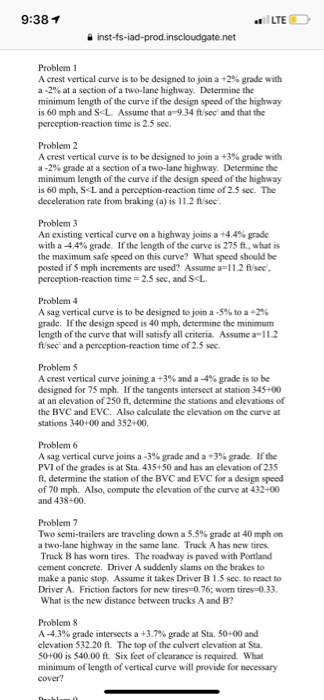9:381 LTE Problem 1 A crest vertical curve is to be designed to join a +2% grade with a-2% at a section of a two-lane highway. Determine the minimum length of the curve if the design speed of the highway is 60 mph and SL. Assume that a-9.34 ftsec' and that the perception-reaction time is 2.5 sec. Problem2 A crest vertical curve is to be designed to join a +3% grade with a-2% grade at a section of a two-lane...

• ### Problem 4 A 500 ft long sag vertical curve passes under a bridge at station 82+45....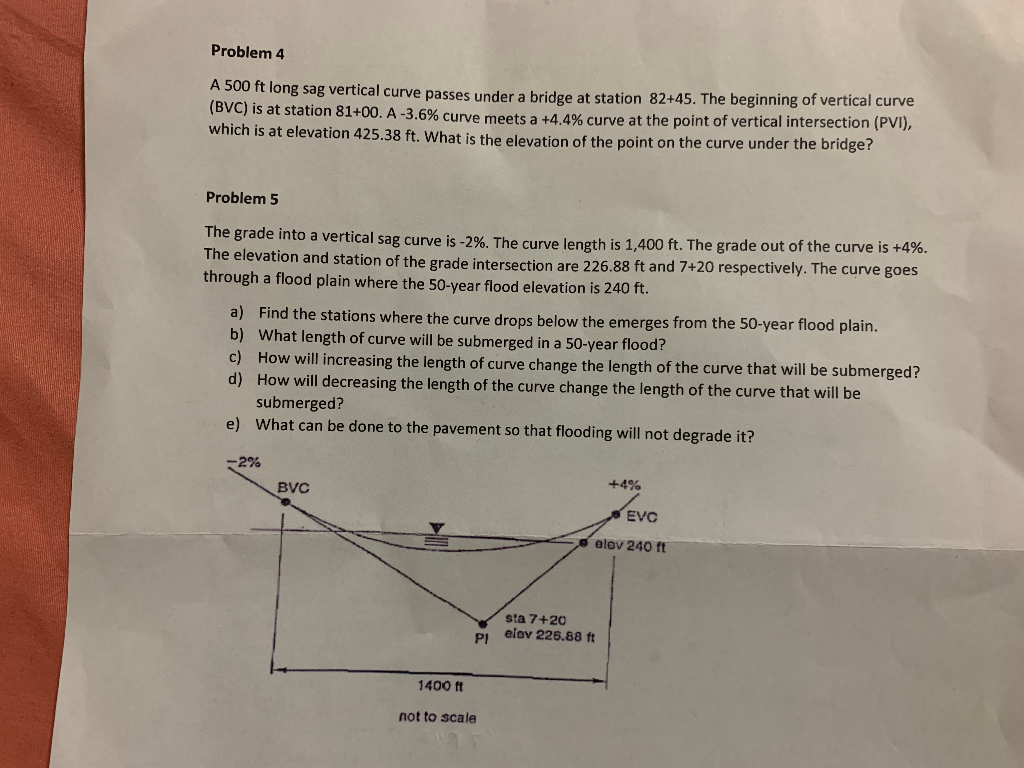Problem 4 A 500 ft long sag vertical curve passes under a bridge at station 82+45. The beginning of vertical curve (BVC) is at station 81+00. A-3.6% curve meets a +4.4% curve at the point of vertical intersection (PVI), which is at elevation 425.38 ft. What is the elevation of the point on the curve under the bridge? Problem 5 The grade into a vertical sag curve is -2%. The curve length is 1,400 ft. The grade out of the...

• ### A vertical parabolic curve is to be used as a crest curve, and it is desired...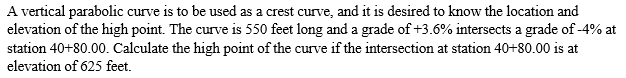A vertical parabolic curve is to be used as a crest curve, and it is desired to know the location and elevation of the high point. The curve is 550 feet long and a grade of +3.6% intersects a grade of-4% at station 40+80.00. Calculate the high point of the curve if the intersection at station 40+80.00 is at elevation of 625 feet.

• ### 2. A crest vertical curve is to be designed by joining a +4% initial tangent with...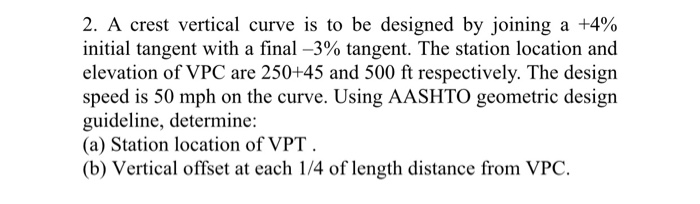2. A crest vertical curve is to be designed by joining a +4% initial tangent with a final -3% tangent. The station location and elevation of VPC are 250+45 and 500 ft respectively. The design speed is 50 mph on the curve. Using AASHTO geometric design guideline, determine: (a) Station location of VPT. (b) Vertical offset at each 1/4 of length distance from VPC.

• ### 6) Given the following vertical curve data: PVI at 18+00; L-500 ft: gi-3.5 %; g,--1.5 %;...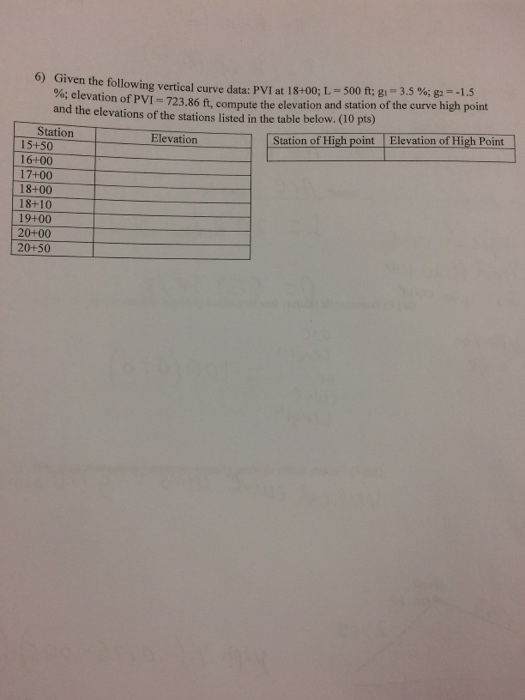6) Given the following vertical curve data: PVI at 18+00; L-500 ft: gi-3.5 %; g,--1.5 %; elevation of PVI-723.86 ft, compute the elevation and station of the curve high point and the elevations of the stations listed in the table below. (10 pts) Station of High point Elevation of High Point Station 15+50 16+00 17+00 18+00 18+10 19+00 20+00 20+50 Elevation

• ### CE 123: HIGHWAY AND STREET DESIGN PROJECT: VERTICAL CRUVES A crest vertical curve is designed for...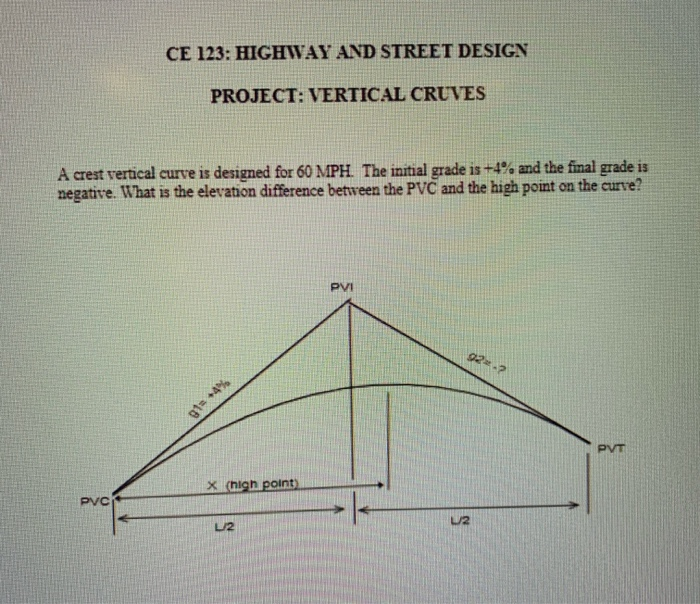CE 123: HIGHWAY AND STREET DESIGN PROJECT: VERTICAL CRUVES A crest vertical curve is designed for 60 MPH. The initial grade is +4% and the final grade is negative. What is the elevation difference between the PVC and the high point on the curve? x (high point) PVC

• ### 9-(10 pts) Stake elevation at each station along the curve, given the below g1-2.5 21.0 Sta ation of BVC 25+50: ength of parabolic curve 500 ft Elevation of BVC 800 ft 9-(10 pts) Stake eleva...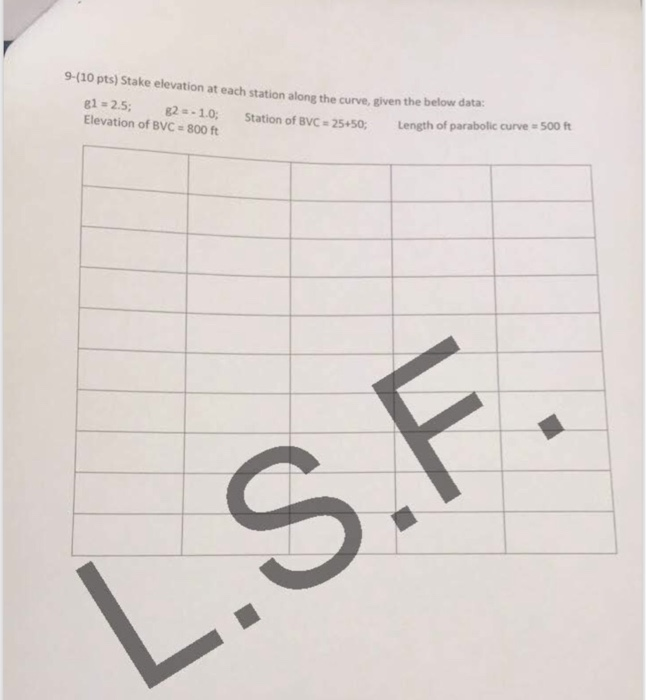9-(10 pts) Stake elevation at each station along the curve, given the below g1-2.5 21.0 Sta ation of BVC 25+50: ength of parabolic curve 500 ft Elevation of BVC 800 ft 9-(10 pts) Stake elevation at each station along the curve, given the below g1-2.5 21.0 Sta ation of BVC 25+50: ength of parabolic curve 500 ft Elevation of BVC 800 ft

• ### 7. A sag vertical curve connects a-4.75% grade and a +3.75% grade. The PVI is at...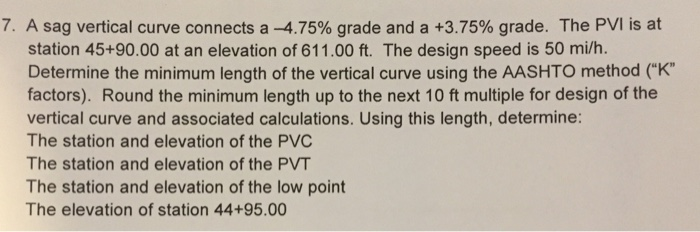7. A sag vertical curve connects a-4.75% grade and a +3.75% grade. The PVI is at station 45+90.00 at an elevation of 611.00 ft. The design speed is 50 mi/h. Determine the minimum length of the vertical curve using the AASHTO method ("K" factors). Round the minimum length up to the next 10 ft multiple for design of the vertical curve and associated calculations. Using this length, determine: The station and elevation of the PVC The station and elevation of...